## Introduction - working with `numpy`

In this session, you will learn about some further techniques in Python. Last week’s session covered the basics of how to code in Python. This week you will look at more data types and tools in Python for advanced data analysis. In particular you will see how Python can handle matrices, and some basic 2d and 3d graphics tools. One of the key packages to enable this is call `numpy`. This package should be available on your standard Anaconda Python installations. One of the key things that `numpy` does is to provide matrix and array data types. Although similar to lists as seen last week, in some ways they are more powerful. The following code demonstrates this:

``````# Firstly import numpy
import numpy as np
# Create two 1D arrays using np.array
x = np.array([5.0,6.0,8.0,10.0])
y = np.array([8.5,8.4,8.1,7.2])
# Note that adding up arrays works element by element
print(x + y)
# and numpy functions also work like this - so eg np.log
# is better with arrays than math.log ``````
``## [ 13.5  14.4  16.1  17.2]``
``````print(np.log(x))
# Two dimensional arrays (ie matrices) are also possible``````
``## [ 1.60943791  1.79175947  2.07944154  2.30258509]``
``````z1 = np.array([[2.0, 3.1, -2.0],[1.0,-1.0,6.3]])
z2 = np.array([[-2.0, 4.7, 3.1],[-1.0,-1.4,1.8]])
print(z1 + z2)``````
``````## [[ 0.   7.8  1.1]
##  [ 0.  -2.4  8.1]]``````
``print(np.sin(z2))``
``````## [[-0.90929743 -0.99992326  0.04158066]
##  [-0.84147098 -0.98544973  0.97384763]]``````

The data types provided by `numpy` are quite similar to the arrays and matrices in R - and are useful for data analysis in the same way. Some of the statistical functions are also provided:

``````# mean, std are supported
print(np.mean(x))``````
``## 7.25``
``````z_score = (x - np.mean(x))/np.std(x)
print(z_score)``````
``## [-1.1717002  -0.65094455  0.39056673  1.43207802]``

You can use these functions in your own functions:

``````def zscore(dat):
return (dat - np.mean(dat)) / np.std(dat)
print(zscore(y))``````
``## [ 0.87831007  0.68313005  0.09759001 -1.65903012]``

## 2D Arrays and Matrices

It is also possible to use `np.mean` and so on with higher dimensional data. Functions that work in this way include `np.mean`, `np.std`, `np.sum`, `np.min` and `np.max`. You can use them to compute values for the entire matrix, or by specifying `axis=0` provide column-wise results, or row-wise results for `axis=1`:

``print(np.mean(z1))``
``## 1.56666666667``
``print(np.sum(z1,axis=0))``
``## [ 3.   2.1  4.3]``
``print(np.min(z1,axis=1))``
``## [-2. -1.]``

Vector and array objects also have - these are basically properties of the objects - such as the number of rows or columns a matrix has. That particular attribute is called :

``print(y.shape)``
``## (4,)``
``print(z2.shape)``
``## (2, 3)``
``print(np.min(z1,axis=0).shape)``
``## (3,)``

The result of these expressions a a - a Python object similar to a list. One useful proprty of tuples is that although it is possible to assign a whole tuple to a variable, it is also possible to do this element by element:

``````num_rows, num_cols = z1.shape
print("Rows =", num_rows, "; Cols=", num_cols)``````
``## Rows = 2 ; Cols= 3``

It is also possible to use slicing on arrays (cf last week). For example, to pick out columns 0 and 1 from `z1` use

``````part_z1 = z1[:,0:2]
print(part_z1)``````
``````## [[ 2.   3.1]
##  [ 1.  -1. ]]``````

Note - a slice expression is needed in both dimensions, hence the `:` in the row dimension - stating that `all` rows are wanted. Also remember that the right-hand value in a slice expression refers to the element `after` the last one to be selected.

Note that for 2D arrays, such as `z1` and `z2`, arithmetic operators `+`, `-`, `*`, `/` and so on work on an element by element basis. Thus, for example:

``````v1 = np.array([[2.0, 3.1, -2.0],[1.0,-1.0,6.3],[2.0,-1.0,3.5]])
v2 = np.array([[-7.3, 6.1, -2.2],[-1.3,1.4,1.7],[-3.0,2.0,-1.0]])
print(v1 * v2)``````
``````## [[-14.6   18.91   4.4 ]
##  [ -1.3   -1.4   10.71]
##  [ -6.    -2.    -3.5 ]]``````

Thus, the `[0,0]` element of the result above is `v1[0,0]` times `v2[0,0]` - this is different from matrix multiplication. `numpy` also offers a data type called `matrix` for which multiplication is actually matrix multiplication:

``````m1 = np.matrix([[2.0, 3.1, -2.0],[1.0,-1.0,6.3],[2.0,-1.0,3.5]])
m2 = np.matrix([[-7.3, 6.1, -2.2],[-1.3,1.4,1.7],[-3.0,2.0,-1.0]])
print(m1 * m2)``````
``````## [[-12.63  12.54   2.87]
##  [-24.9   17.3  -10.2 ]
##  [-23.8   17.8   -9.6 ]]``````

## Data via `pandas`

Another helpful Python package is `pandas`. This provides a new data type which is very similar to an R **data frame*. This package is used for a number of data manipulation tasks - one of the most practical is its ability to read in and manipulate data stored in `csv` files. For this part of the practical, you will need some data relating to house prices. Currently this is available in the `NCG603` directory on Dropbox, which can be downloaded from https://www.dropbox.com/s/2bfz210216dw6cw/hpdemo.csv?dl=0. If you open this with a text editor, the first few lines look like this:

``````"ID","east","north","price","fl_area"
"1",523800,179700,107000,50
"2",533200,170900,55500,66
"3",514600,175800,103000,90
"4",516000,171000,187000,125
"5",533700,169200,43000,50``````

Respectively, the columns are:

Variable Description
ID An ID number for each house
east The easting (x-coordinate) of the house
north The northing (y-coordinate) of the house
price The sale price of the house
fl_area The floor area of the house

The `pandas` package has a useful function for reading `csv` files. Make sure the house price file is in the folder you are working in, and then enter:

``````import pandas as pd
print(hp)``````
``````##         ID    east   north   price  fl_area
## 0        1  523800  179700  107000       50
## 1        2  533200  170900   55500       66
## 2        3  514600  175800  103000       90
## 3        4  516000  171000  187000      125
## 4        5  533700  169200   43000       50
## 5        6  547900  189600   69995       95
## 6        7  531900  189900   80000       79
## 7        8  514600  190300   57500       44
## 8        9  525700  172900  104000       68
## 9       10  536700  188100   69000       87
## 10      11  529000  171300   88000       76
## 11      12  523900  191200  123000      107
## 12      13  546800  169600   83000       92
## 13      14  547100  187600   52995       53
## 14      15  531900  185800  255000      146
## 15      16  528700  173400  185000      125
## 16      17  529200  164400  152000      157
## 17      18  525000  190200   85000       79
## 18      19  514600  189300  120000      108
## 19      20  524200  174600  135000       91
## 20      21  516300  176700  143000      100
## 21      22  505100  183400   59950       39
## 22      23  523800  184600  285000      198
## 23      24  526400  177100  272500      155
## 24      25  532800  167200   73950       87
## 25      26  533700  166500   67500       59
## 26      27  514200  172200  174000      114
## 27      28  541200  178200   65000       92
## 28      29  538400  191500   50300       61
## 29      30  539900  190200   63200       62
## ...    ...     ...     ...     ...      ...
## 1375  1376  528000  176400   52000       83
## 1376  1377  539300  169400  142000      127
## 1377  1378  537100  182700   70000       60
## 1378  1379  531200  194600  243000      129
## 1379  1380  527900  166100   58000       48
## 1380  1381  522900  168500  139950      120
## 1381  1382  518800  163600  125000       98
## 1382  1383  522200  177600  134000       70
## 1383  1384  526700  175100   85000       46
## 1384  1385  528900  194900   99000       96
## 1385  1386  526700  165900   77000       80
## 1386  1387  527000  171600   76000       80
## 1387  1388  538700  185600   64000       76
## 1388  1389  540900  173700   64000       77
## 1389  1390  526000  173800   59999       52
## 1390  1391  552000  190400  246000      155
## 1391  1392  536000  169200  122000      127
## 1392  1393  532000  172200   79000       75
## 1393  1394  536600  165300  139950      110
## 1394  1395  527600  193300   65000       54
## 1395  1396  520000  186900  250000      136
## 1396  1397  511300  176400   66950      105
## 1397  1398  522400  166400  119950       85
## 1398  1399  528300  175900  151500       75
## 1399  1400  528800  171300  230000      178
## 1400  1401  515600  173100   68500       44
## 1401  1402  513200  186500   58500       59
## 1402  1403  542900  189500  247000      185
## 1403  1404  524900  185300  153000       96
## 1404  1405  522000  185400  146250      111
##
## [1405 rows x 5 columns]``````

You can access the contents of the columns in the same way as you access items in dictionaries:

``print(hp['price'])``
``````## 0       107000
## 1        55500
## 2       103000
## 3       187000
## 4        43000
## 5        69995
## 6        80000
## 7        57500
## 8       104000
## 9        69000
## 10       88000
## 11      123000
## 12       83000
## 13       52995
## 14      255000
## 15      185000
## 16      152000
## 17       85000
## 18      120000
## 19      135000
## 20      143000
## 21       59950
## 22      285000
## 23      272500
## 24       73950
## 25       67500
## 26      174000
## 27       65000
## 28       50300
## 29       63200
##          ...
## 1375     52000
## 1376    142000
## 1377     70000
## 1378    243000
## 1379     58000
## 1380    139950
## 1381    125000
## 1382    134000
## 1383     85000
## 1384     99000
## 1385     77000
## 1386     76000
## 1387     64000
## 1388     64000
## 1389     59999
## 1390    246000
## 1391    122000
## 1392     79000
## 1393    139950
## 1394     65000
## 1395    250000
## 1396     66950
## 1397    119950
## 1398    151500
## 1399    230000
## 1400     68500
## 1401     58500
## 1402    247000
## 1403    153000
## 1404    146250
## Name: price, Length: 1405, dtype: int64``````

This lists all of the items in the column `price`. Sometimes rescaling is useful - particularly to obtain ‘neater’ axes.

``print(hp['price']/1000)``
``````## 0       107.000
## 1        55.500
## 2       103.000
## 3       187.000
## 4        43.000
## 5        69.995
## 6        80.000
## 7        57.500
## 8       104.000
## 9        69.000
## 10       88.000
## 11      123.000
## 12       83.000
## 13       52.995
## 14      255.000
## 15      185.000
## 16      152.000
## 17       85.000
## 18      120.000
## 19      135.000
## 20      143.000
## 21       59.950
## 22      285.000
## 23      272.500
## 24       73.950
## 25       67.500
## 26      174.000
## 27       65.000
## 28       50.300
## 29       63.200
##          ...
## 1375     52.000
## 1376    142.000
## 1377     70.000
## 1378    243.000
## 1379     58.000
## 1380    139.950
## 1381    125.000
## 1382    134.000
## 1383     85.000
## 1384     99.000
## 1385     77.000
## 1386     76.000
## 1387     64.000
## 1388     64.000
## 1389     59.999
## 1390    246.000
## 1391    122.000
## 1392     79.000
## 1393    139.950
## 1394     65.000
## 1395    250.000
## 1396     66.950
## 1397    119.950
## 1398    151.500
## 1399    230.000
## 1400     68.500
## 1401     58.500
## 1402    247.000
## 1403    153.000
## 1404    146.250
## Name: price, Length: 1405, dtype: float64``````

## Basic Statistical Graphics

Having read in this data, we can start to analysis and visualise it in Python. Firstly, to visualise it the `pyplot` package (a sub-package of `matplotlib`)1 can be used - this produces Python plots that look quite like ones that MATLAB produces.

``````import matplotlib.pyplot as pl
# Try to draw a histogram
pl.hist(hp['price'])
pl.show()``````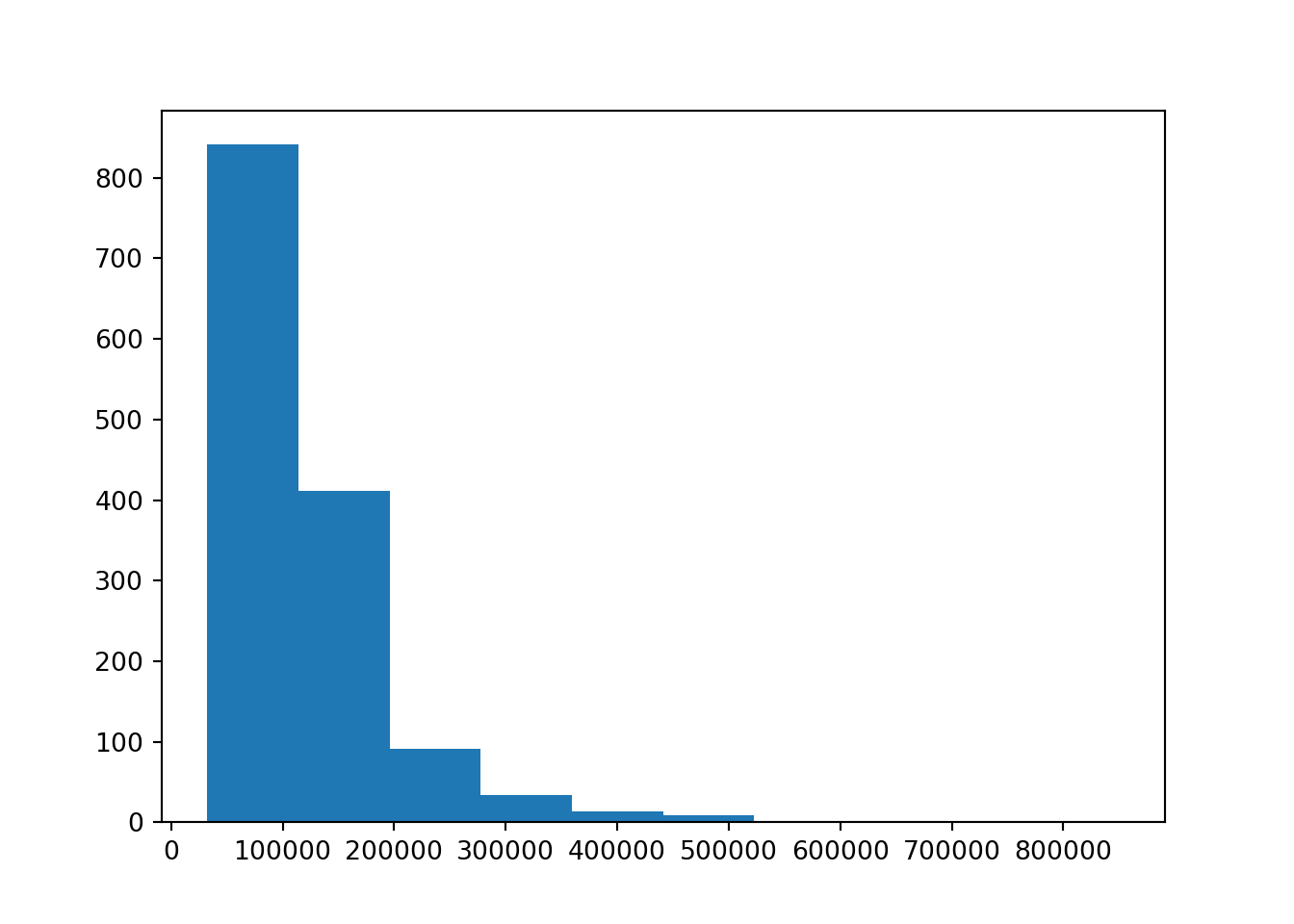You will see that at the `pl.hist` stage no graphic appears. `pylab` works by building up the layers in a image, and then when everything in place, using the `show` function to actually create the plot.

This would perhaps look better if the prices were in thousands of pounds and some titles and labels were provided. A nicer result is obtained using:

``````# Try to draw a neater histogram
pl.close()
pl.hist(hp['price']/1000)
pl.xlabel('Price (1000s Pounds)')
pl.ylabel('Frequency')
pl.title('London House Prices')
pl.show()``````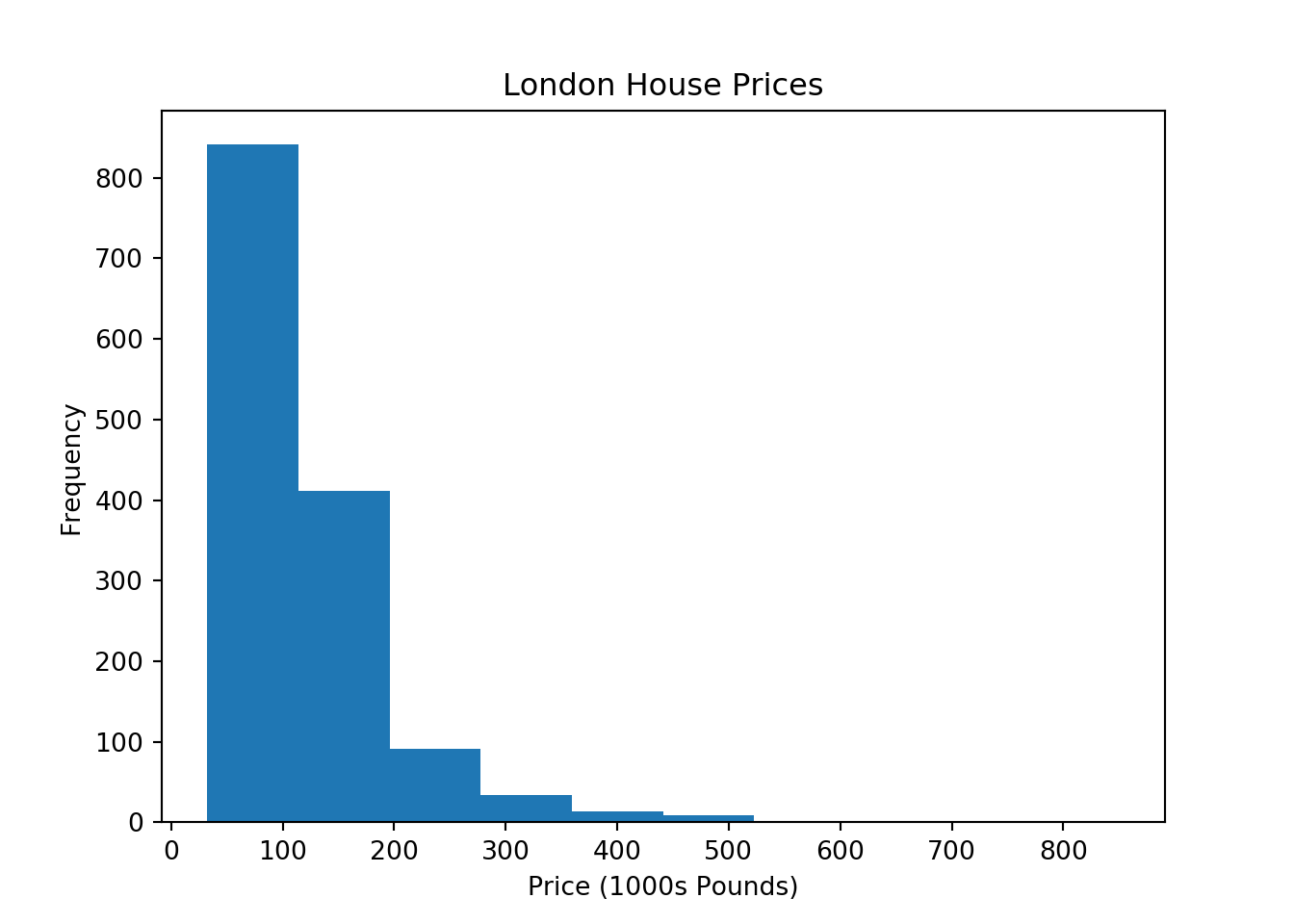Note the way the graphic is ‘built up’ by putting the data, and then various text labels on step by step. Finally, the `show` command is added, to draw the completed graphic. The initial `pl.close()` function closes the previous plot (otherwise the new plot would be overplotted on the original axes).

You can use some of the `numpy` operations on data frames - for example it might be useful to see the distribution of log house prices - this sometimes makes the distribution more like a log normal.

``````# histogram of log house price
pl.close()
pl.hist(np.log10(hp['price']))
pl.xlabel('Logarithm of Price (1000s Pounds)')
pl.ylabel('Frequency')
pl.title('Logged house prices')
pl.show()``````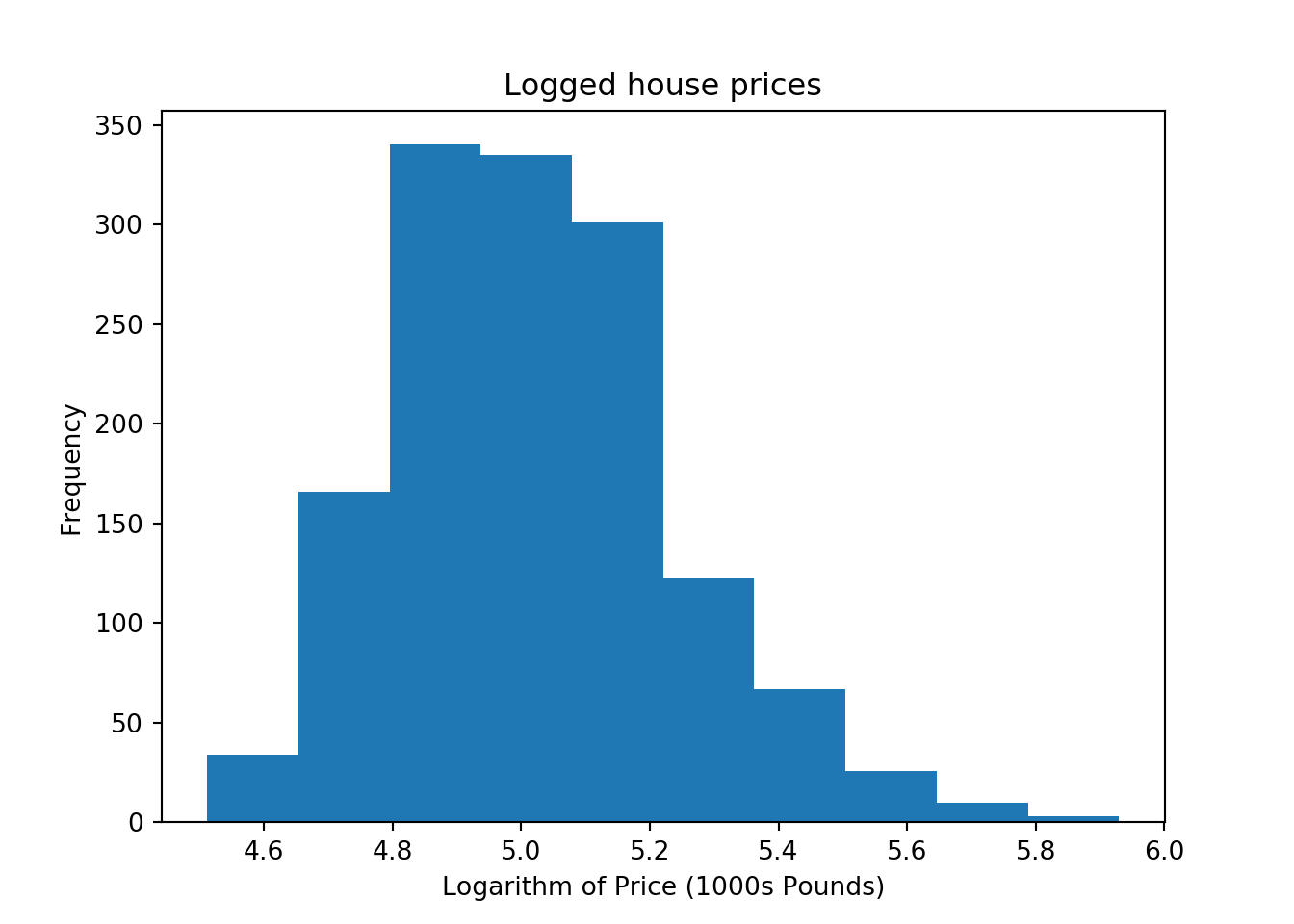Here the function `log10` is used - taking logs to the base 10 of the prices - so that \(\log_{10}(10,000) = 4\), \(\log_{10}(100,000) = 5\) and \(\log_{10}(1,000,000) = 6\).

It is also possible to create scatter plots - using `scatter`:

``````pl.close()
pl.scatter(hp['fl_area'],hp['price']/1000,color='r',marker='.')
pl.xlabel('Floor Area (sq. metres)')
pl.ylabel('Price (1000s Pounds)')
pl.title('House Price v. Floor Area (London)')
pl.show()``````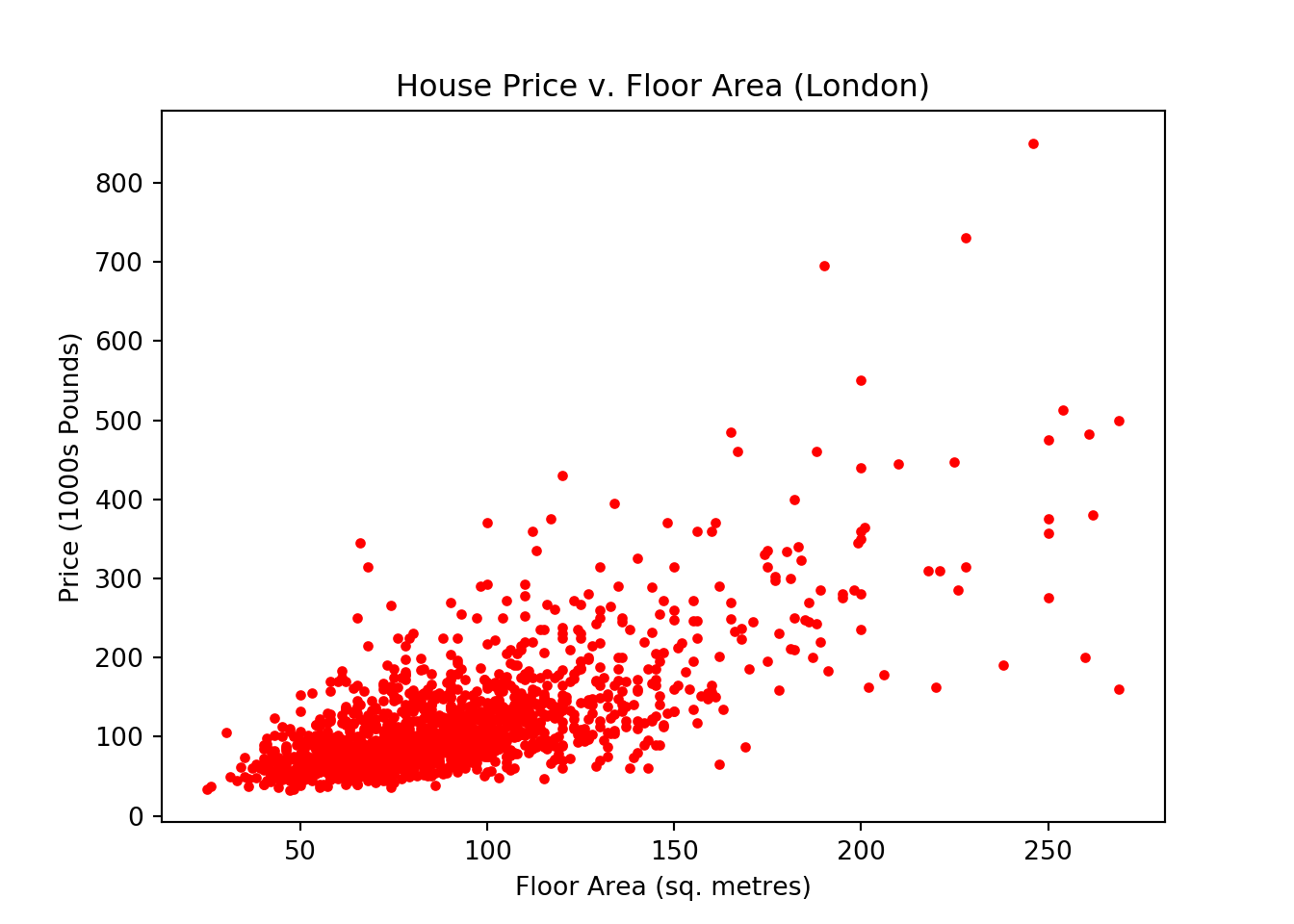Interestingly, although there is some linkage, the relationship is not excessively strong. Note that the dot density is quite high in the lower left hand corner, so it is hard to see relative numbers of points. One way of addressing this is to use semi-transparent points. In the `scatter` function, the colour was specified as `r` (for red), but it is also possible to denote colour as a red, green, blue, transparency 4-tuple. In the case of the three colours, levels range from 0 to 1 - for transparancy the level also runs from 0 to 1, with 0 being completely transparent, and 1 not transparent at all.

``````pl.close()
pl.scatter(hp['fl_area'],hp['price']/1000,color=(1,0,0,0.1),marker='.')
pl.xlabel('Floor Area (sq. metres)')
pl.ylabel('Price (1000s Pounds)')
pl.title('House Price v. Floor Area (London)')
pl.grid(True)
pl.show()``````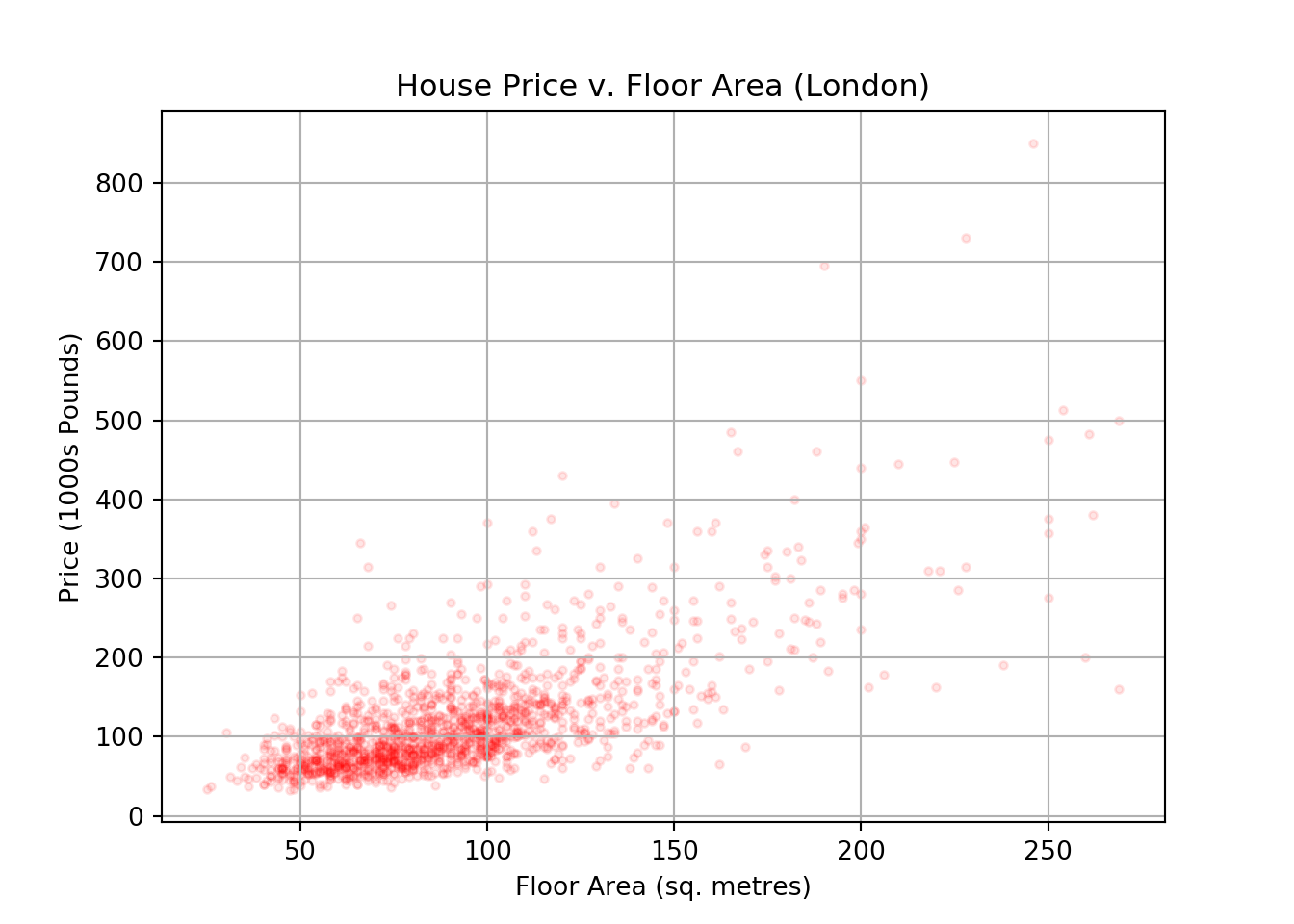Note a grid was also added here. The log transform is also interesting here. Suppose we take logs of both the price and the floor area and plot the result:

``````pl.close()
pl.scatter(np.log10(hp['fl_area']),np.log10(hp['price']),color='r',marker='.')
pl.xlabel('Log Floor Area (sq. metres)')
pl.ylabel('Log Price')
pl.title('House Price v. Floor Area (London) - all data log transformed')
pl.grid(True)
pl.show()``````